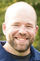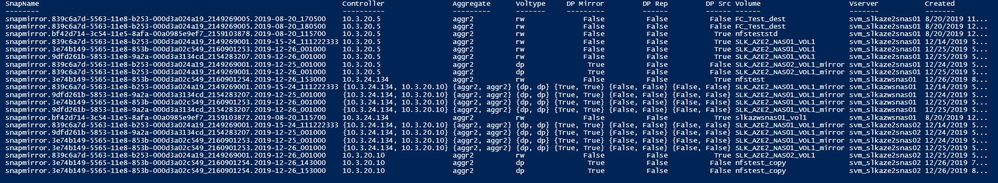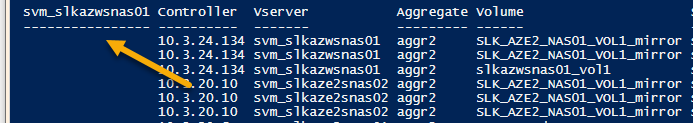Microsoft Virtualization Discussions

## Displaying output from multiple commands get-ncvol/get-ncsnapshot2,927 Views

I'm trying to find some orphaned snapmirrors by searching multiple controllers and filtering for snapshots using get-ncsnapshot with "snapmirror" in the name.  This is working as expected.  In building a query for a report, I want volume attributes on the volume contained in the get-ncvol command,  { (Get-NcVol \$_.Volume).Aggregate }} for example.  This works as expected however; when I try to include an attribute that's nested  "@{l = "Voltype"; e = { (Get-NcVol \$_.volume).VolumeIdAttributes.Type }}," .  The output groups by the volume name.

Note: Any recommandations welcome.

#Connect to Controllers
foreach (\$i in @(\$controllers)) {
Connect-NcController \$i

}

foreach (\$i in @(\$controllers)) {

}

\$date = (Get-Date -Format yy-MM-dd)
\$ExcelName = \$NcController + '_' + \$date + '.xlsx'
\$Days = 7
\$Daysback = \$Days/-1
#
#Snapshot Logic
#
\$Snapshots = Get-NcSnapshot |
Where-Object {
\$_.Name -match 'snapmirror'

}

\$reportData = \$Snapshots | Select-Object @{l = "Controller"; e = { (Get-NcVol \$_.Volume).NcController }},
@{l = "Vserver"; e = {\$_.Vserver}},
@{l = "Aggregate"; e = { (Get-NcVol \$_.Volume).Aggregate }},
@{l = "Volume"; e = { (Get-NcVol \$_.volume).Name }},
@{l = "SnapName"; e = {\$_.Name}},
@{l = "Created"; e = {\$_.Created}},
@{l = "Dependency"; e = {\$_.Dependency}},
@{l = "State"; e = {\$_.State}},
@{l = "Voltype"; e = { (Get-NcVol \$_.volume).VolumeIdAttributes.Type }},
@{l = "Cumulative_GB"; e = {\$([Math]::Round(\$_.Cumulative / 1GB, 2)).ToString()}},
@{l = "Total_GB"; e = {\$([Math]::Round(\$_.Total / 1GB, 2)).ToString() }}

\$reportData | out-gridview

1 ACCEPTED SOLUTION2,746 Views

Thanks for the output. I reworked it a bit, how's this?

```# Create empty array to store snapshots from each controller
\$Snapshots = @()
# Define date and snapshot age variables
\$date = (Get-Date -Format yy-MM-dd)
\$Days = 7
\$Daysback = \$Days/-1
# Connect to controllers
foreach (\$controller in @(\$controllers)) {
Connect-NcController \$controller
# Collect desired snapshots from controllers and store in variable
\$Snapshots += Get-NcSnapshot -Controller \$_ | Where-Object {\$_.Name -match 'snapmirror' -and \$_.Created -lt (Get-Date).AddDays(\$Daysback)}
} #foreach
\$reportData = foreach (\$snapshot in \$snapshots) {
Get-NcVol \$Snapshot.Volume -Controller \$snapshot.NcController | Select-Object @{l = "Controller"; e = {\$(\$_.NcController.Name)}},
@{l = "Vserver"; e = {\$Snapshot.Vserver}},
@{l = "Aggregate"; e = {\$_.Aggregate}},
@{l = "Volume"; e = {\$_.Name}},
@{l = "SnapName"; e = {\$Snapshot.Name}},
@{l = "Created"; e = {\$Snapshot.Created}},
@{l = "DP Mirror"; e = { \$(\$_.VolumeMirrorAttributes.IsDataProtectionMirror) }},
@{l = "DP Rep"; e = { \$(\$_.VolumeMirrorAttributes.IsReplicaVolume) }},
@{l = "DP Src"; e = { \$(\$_.VolumeMirrorAttributes.IsSnapmirrorSource) }},
@{l = "Dependency"; e = {\$Snapshot.Dependency}},
@{l = "State"; e = {\$Snapshot.State}},
@{l = "Voltype"; e = {\$(\$_.VolumeIdAttributes.Type)}},
@{l = "Cumulative_GB"; e = {\$([Math]::Round(\$Snapshot.Cumulative / 1GB, 2)).ToString()}},
@{l = "Total_GB"; e = {\$([Math]::Round(\$Snapshot.Total / 1GB, 2)).ToString()}}
} #foreach
\$reportData | Out-GridView```

It returned good data in my lab across 3 controllers without the erroneous array creation in those properties (and took about 10 seconds to run across 150 snapshots).

Hope that helps!

5 REPLIES 52,826 Views

What version of PS and the NetApp PSTK are you using? Can you show a screenshot of the output that explains what you're seeing compared to how you'd like the data to be formatted? I ran your code against a couple of test controllers (once with the highlighted red line present and once with it commented out) and it appeared identical, aside from the VolType column, of course.2,813 Views

PSTK v9.6

Without get-ncvol commands
\$reportData = \$Snapshots | Select-Object @{l = "SnapName"; e = {\$_.Name}},
#@{l = "Controller"; e = { (Get-NcVol \$_.Volume).NcController }},
#@{l = "Aggregate"; e = { (Get-NcVol \$_.Volume).Aggregate }},
#@{l = "Voltype"; e = { (Get-NcVol \$_.volume).VolumeIdAttributes.Type }},
#@{l = "DP Mirror"; e = { (Get-NcVol \$_.Volume).VolumeMirrorAttributes.IsDataProtectionMirror }},
#@{l = "DP Rep"; e = { (Get-NcVol \$_.Volume).VolumeMirrorAttributes.IsReplicaVolume }},
#@{l = "DP Src"; e = { (Get-NcVol \$_.Volume).VolumeMirrorAttributes.IsSnapmirrorSource }},
@{l = "Volume"; e = {\$_.Volume }},
@{l = "Vserver"; e = {\$_.Vserver}},
@{l = "Created"; e = {\$_.Created}},
@{l = "Dependency"; e = {\$_.Dependency}},
@{l = "Cumulative_GB"; e = {\$([Math]::Round(\$_.Cumulative / 1GB, 2)).ToString()}},
@{l = "Total_GB"; e = {\$([Math]::Round(\$_.Total / 1GB, 2)).ToString() }}
\$reportData | Format-Table\$reportData = \$Snapshots | Select-Object @{l = "SnapName"; e = {\$_.Name}},
@{l = "Controller"; e = { (Get-NcVol \$_.Volume).NcController }},
@{l = "Aggregate"; e = { (Get-NcVol \$_.Volume).Aggregate }},
@{l = "Voltype"; e = { (Get-NcVol \$_.volume).VolumeIdAttributes.Type }},
@{l = "DP Mirror"; e = { (Get-NcVol \$_.Volume).VolumeMirrorAttributes.IsDataProtectionMirror }},
@{l = "DP Rep"; e = { (Get-NcVol \$_.Volume).VolumeMirrorAttributes.IsReplicaVolume }},
@{l = "DP Src"; e = { (Get-NcVol \$_.Volume).VolumeMirrorAttributes.IsSnapmirrorSource }},
@{l = "Volume"; e = {\$_.Volume }},
@{l = "Vserver"; e = {\$_.Vserver}},
@{l = "Created"; e = {\$_.Created}},
@{l = "Dependency"; e = {\$_.Dependency}},
@{l = "Cumulative_GB"; e = {\$([Math]::Round(\$_.Cumulative / 1GB, 2)).ToString()}},
@{l = "Total_GB"; e = {\$([Math]::Round(\$_.Total / 1GB, 2)).ToString() }}
\$reportData | Format-TableIt seems like it's building an array each time a get-ncvol is issued and returning the first value .  When usin 'export-excel', only the first entry is displayed and refer to the controler field, defaults to the .134 controller when the value should exist on all three controllers:

Condensed view:

 SnapName Controller Aggregate Voltype DP Mirror DP Rep DP Src Volume Vserver Created Dependency Cumulative_GB Total_GB snapmirror.839c6a7d-5563-11e8-b253-000d3a024a19_2149269001.2019-15-24_111222333 10.3.20.5 aggr2 rw FALSE FALSE TRUE SLK_AZE2_NAS01_VOL1 svm_slkaze2snas01 12/14/2019 17:15 0 0 snapmirror.839c6a7d-5563-11e8-b253-000d3a024a19_2149269001.2019-15-24_111222333 10.3.24.134 aggr2 dp TRUE FALSE FALSE SLK_AZE2_NAS01_VOL1_mirror svm_slkazwsnas01 12/14/2019 17:15 0 0 snapmirror.839c6a7d-5563-11e8-b253-000d3a024a19_2149269001.2019-15-24_111222333 10.3.24.134 aggr2 dp TRUE FALSE FALSE SLK_AZE2_NAS01_VOL1_mirror svm_slkaze2snas02 12/14/2019 17:15 0 0

Also noting, when running the get-ncvol commands, it takes a long time to execute.2,747 Views

Thanks for the output. I reworked it a bit, how's this?

```# Create empty array to store snapshots from each controller
\$Snapshots = @()
# Define date and snapshot age variables
\$date = (Get-Date -Format yy-MM-dd)
\$Days = 7
\$Daysback = \$Days/-1
# Connect to controllers
foreach (\$controller in @(\$controllers)) {
Connect-NcController \$controller
# Collect desired snapshots from controllers and store in variable
\$Snapshots += Get-NcSnapshot -Controller \$_ | Where-Object {\$_.Name -match 'snapmirror' -and \$_.Created -lt (Get-Date).AddDays(\$Daysback)}
} #foreach
\$reportData = foreach (\$snapshot in \$snapshots) {
Get-NcVol \$Snapshot.Volume -Controller \$snapshot.NcController | Select-Object @{l = "Controller"; e = {\$(\$_.NcController.Name)}},
@{l = "Vserver"; e = {\$Snapshot.Vserver}},
@{l = "Aggregate"; e = {\$_.Aggregate}},
@{l = "Volume"; e = {\$_.Name}},
@{l = "SnapName"; e = {\$Snapshot.Name}},
@{l = "Created"; e = {\$Snapshot.Created}},
@{l = "DP Mirror"; e = { \$(\$_.VolumeMirrorAttributes.IsDataProtectionMirror) }},
@{l = "DP Rep"; e = { \$(\$_.VolumeMirrorAttributes.IsReplicaVolume) }},
@{l = "DP Src"; e = { \$(\$_.VolumeMirrorAttributes.IsSnapmirrorSource) }},
@{l = "Dependency"; e = {\$Snapshot.Dependency}},
@{l = "State"; e = {\$Snapshot.State}},
@{l = "Voltype"; e = {\$(\$_.VolumeIdAttributes.Type)}},
@{l = "Cumulative_GB"; e = {\$([Math]::Round(\$Snapshot.Cumulative / 1GB, 2)).ToString()}},
@{l = "Total_GB"; e = {\$([Math]::Round(\$Snapshot.Total / 1GB, 2)).ToString()}}
} #foreach
\$reportData | Out-GridView```

It returned good data in my lab across 3 controllers without the erroneous array creation in those properties (and took about 10 seconds to run across 150 snapshots).

Hope that helps!2,738 Views

Nicely done!  Everything appears to be working.  Any idea what's creating this empty column?2,735 Views

Oops, I had an extra property in the Select-Object statement, I fixed it in my code above.